Introduction To Electric Circuits - 9 Edition - Chapter 7.2 - Problem P7.2-3
Register Now

Join StudySoup

Get Full Access to Introduction To Electric Circuits - 9 Edition - Chapter 7.2 - Problem P7.2-39781118477502

# Solved: The voltage v(t) across a capacitor and current i(t) in that capacitor adhere to

Introduction to Electric Circuits | 9th Edition

Problem P7.2-3

The voltage v(t) across a capacitor and current i(t) in that capacitor adhere to the passive convention. Determine the capacitance when the voltage is v t 12 cos 500t 45 V and the current is i t 3 cos 500t 45 mA.

Accepted Solution
Step-by-Step Solution:

Step 1 of 3

Current through a capacitor is equal to: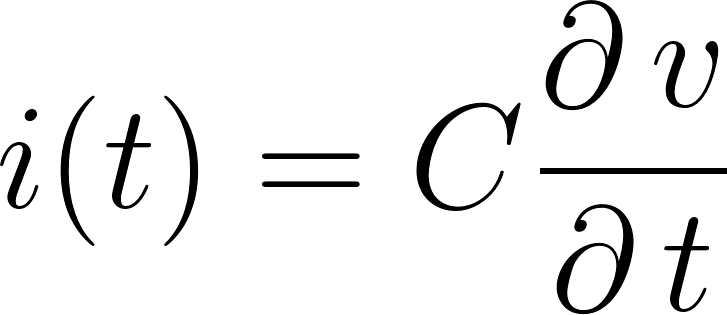Solving for: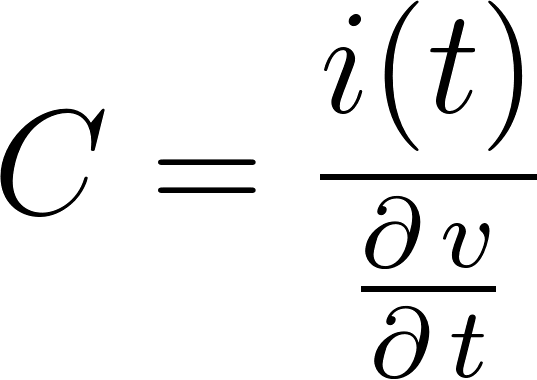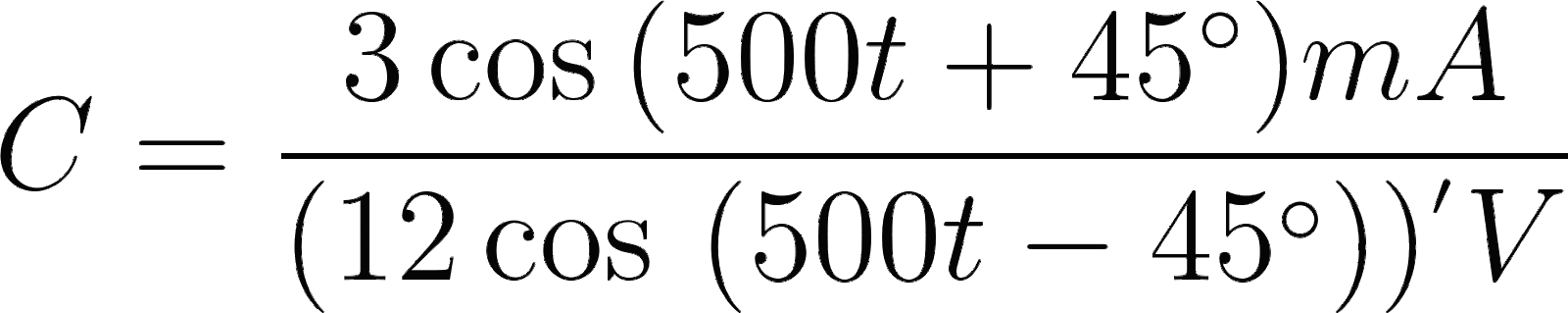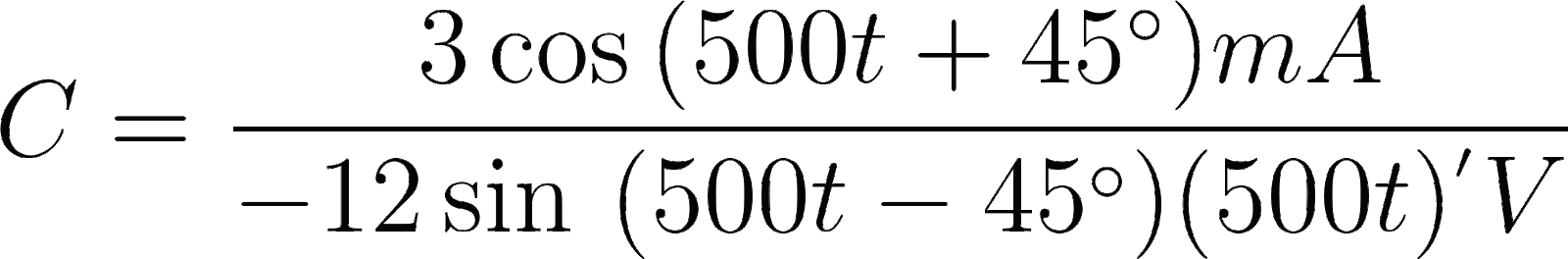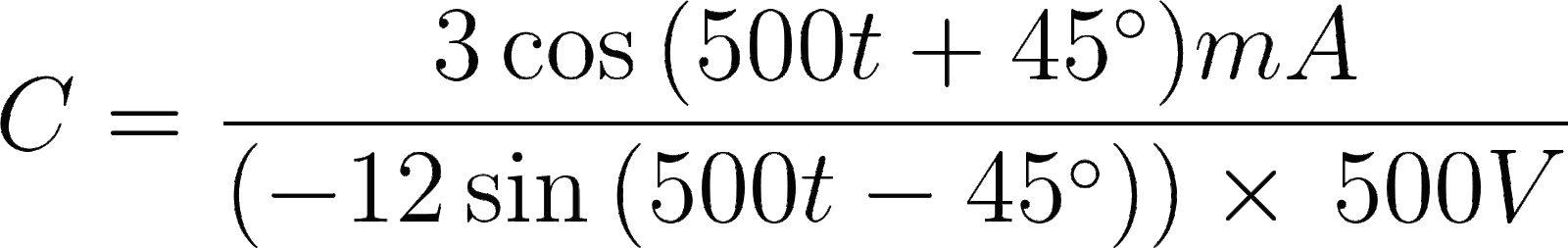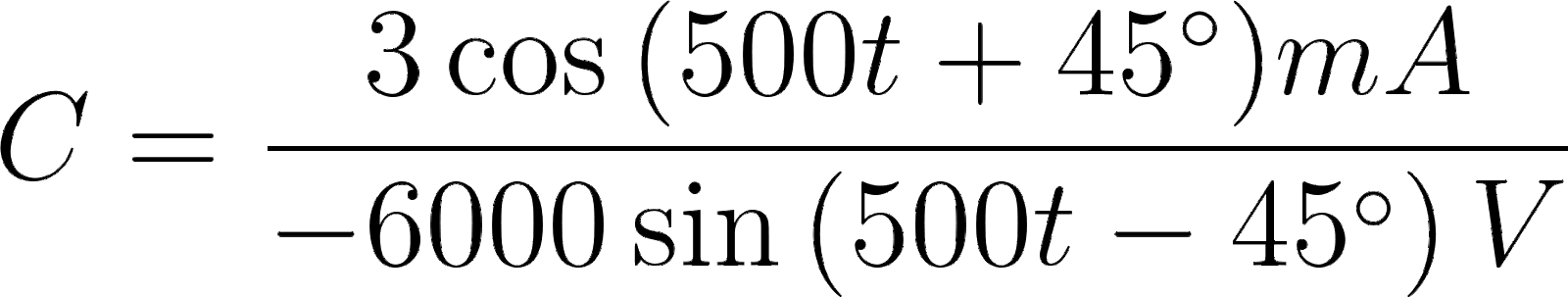(1)

###### Chapter 7.2, Problem P7.2-3 is Solved

Step 2 of 3

Step 3 of 3

Unlock Textbook Solution